# monopoleTopHat

Create capacitively loaded monopole antenna over rectangular ground plane

## Description

The `monopoleTopHat` object is a top-hat monopole antenna mounted over a rectangular ground plane. The monopole always connects with the center of top hat. The top hat builds up additional capacitance to ground within the structure. This capacitance reduces the resonant frequency of the antenna without increasing the size of the element.

The width of the monopole is related to the diameter of an equivalent cylindrical monopole by the expression

`$w=2d=4r$`

where:

• d is the diameter of equivalent cylindrical monopole

• r is the radius of equivalent cylindrical monopole.

For a given cylinder radius, use the `cylinder2strip` utility function to calculate the equivalent width. The default top-hat monopole is center-fed. The feed point coincides with the origin. The origin is located on the xy- plane.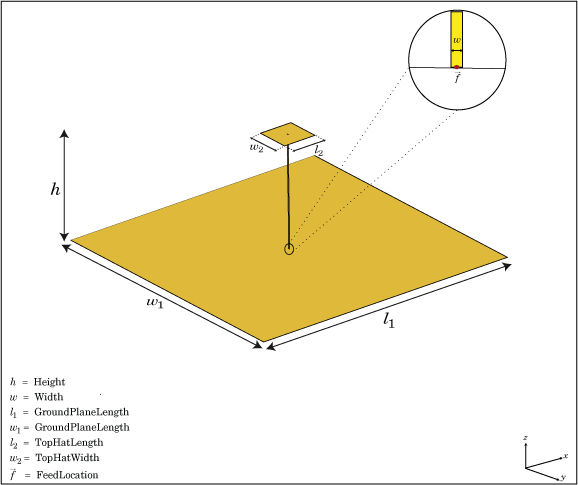## Creation

### Syntax

``mth = monopoleTopHat``
``mth = monopoleTopHat(Name,Value)``

### Description

example

``` `mth = monopoleTopHat` creates a capacitively loaded monopole antenna over a rectangular ground plane.```
``` `mth = monopoleTopHat(Name,Value)` creates a capacitively loaded monopole antenna with additional properties specified by one or more name-value pair arguments. `Name` is the property name and `Value` is the corresponding value. You can specify several name-value pair arguments in any order as `Name1`, `Value1`,`...`, `NameN`, `ValueN`. Properties not specified retain their default values.```

## Properties

expand all

Monopole height, specified as a scalar in meters. By default, the height is chosen for an operating frequency of 75 MHz.

Example: `'Height',3`

Data Types: `double`

Monopole width, specified as a scalar in meters.

Note

Monopole width should be less than `'Height'`/4 and greater than `'Height'`/1001. 

Example: `'Width',0.05`

Data Types: `double`

Ground plane length along x-axis, specified as a scalar in meters. Setting `'GroundPlaneLength'` to `Inf`, uses the infinite ground plane technique for antenna analysis.

Example: `'GroundPlaneLength',4`

Data Types: `double`

Ground plane width along y-axis, specified as a scalar in meters. Setting `'GroundPlaneWidth'` to `Inf`, uses the infinite ground plane technique for antenna analysis.

Example: `'GroundPlaneWidth',2.5`

Data Types: `double`

Top hat length along x-axis, specified as a scalar in meters.

Example: `'TopHatLength',4`

Data Types: `double`

Top hat width along y-axis, specified as a scalar in meters.

Example: `'TopHatWidth',4`

Data Types: `double`

Signed distance from center along length and width of ground plane, specified as a two-element vector.

Example: `'FeedOffset',[2 1]`

Data Types: `double`

Type of dielectric material used as the substrate, specified as a dielectric object. You can also specify multiple dielectric layers. When creating multiple dielectric layers, in the `dielectric` function, specify the name, thickness, loss tangent, and relative permittivity of each layer. For more information, see `dielectric`. For more information on dielectric substrate meshing, see Meshing.

Example: ```d = dielectric('FR4'); mth = monopoleTopHat('Substrate',d)```

Example: ```d = dielectric('FR4'); mth = MonopoleTopHat; mth.Substrate = d```

Example: ```d = dielectric('Name',{'FR4','Teflon'},'Thickness',[0.5 0.5],'LossTangent',[0.002 0.002],'EpsilonR',[4.8 2.1]); mth = monopoleTopHat('Substrate',d)```

Type of the metal used as a conductor, specified as a metal material object. You can choose any metal from the `MetalCatalog` or specify a metal of your choice. For more information, see `metal`. For more information on metal conductor meshing, see Meshing.

Example: ```m = metal('Copper'); 'Conductor',m```

Example: ```m = metal('Copper'); ant.Conductor = m```

Lumped elements added to the antenna feed, specified as a lumped element object. For more information, see `lumpedElement`.

Example: `'Load',lumpedelement`. `lumpedelement` is the object for the load created using `lumpedElement`.

Example: ```mth.Load = lumpedElement('Impedance',75)```

Tilt angle of the antenna, specified as a scalar or vector with each element unit in degrees. For more information, see Rotate Antennas and Arrays.

Example: `Tilt=90`

Example: `Tilt=[90 90]`,`TiltAxis=[0 1 0;0 1 1]` tilts the antenna at 90 degrees about the two axes defined by the vectors.

Note

The `wireStack` antenna object only accepts the dot method to change its properties.

Data Types: `double`

Tilt axis of the antenna, specified as:

• Three-element vector of Cartesian coordinates in meters. In this case, each coordinate in the vector starts at the origin and lies along the specified points on the X-, Y-, and Z-axes.

• Two points in space, each specified as three-element vectors of Cartesian coordinates. In this case, the antenna rotates around the line joining the two points in space.

• A string input describing simple rotations around one of the principal axes, 'X', 'Y', or 'Z'.

Example: `TiltAxis=[0 1 0]`

Example: `TiltAxis=[0 0 0;0 1 0]`

Example: `TiltAxis = 'Z'`

Data Types: `double`

## Object Functions

 `show` Display antenna, array structures or shapes `info` Display information about antenna or array `axialRatio` Axial ratio of antenna `beamwidth` Beamwidth of antenna `charge` Charge distribution on antenna or array surface `current` Current distribution on antenna or array surface `design` Design prototype antenna or arrays for resonance around specified frequency `efficiency` Radiation efficiency of antenna `EHfields` Electric and magnetic fields of antennas; Embedded electric and magnetic fields of antenna element in arrays `impedance` Input impedance of antenna; scan impedance of array `mesh` Mesh properties of metal, dielectric antenna, or array structure `meshconfig` Change mesh mode of antenna structure `optimize` Optimize antenna or array using SADEA optimizer `pattern` Radiation pattern and phase of antenna or array; Embedded pattern of antenna element in array `patternAzimuth` Azimuth pattern of antenna or array `patternElevation` Elevation pattern of antenna or array `rcs` Calculate and plot radar cross section (RCS) of platform, antenna, or array `returnLoss` Return loss of antenna; scan return loss of array `sparameters` Calculate S-parameter for antenna and antenna array objects `vswr` Voltage standing wave ratio of antenna

## Examples

collapse all

Create and view a top-hat monopole with 1 m length, 0.01 m width, groundplane dimensions 2mx2m and top hat dimensions 0.25mx0.25m.

`th = monopoleTopHat`
```th = monopoleTopHat with properties: Height: 1 Width: 0.0100 GroundPlaneLength: 2 GroundPlaneWidth: 2 TopHatLength: 0.2500 TopHatWidth: 0.2500 Substrate: [1x1 dielectric] FeedOffset: [0 0] Conductor: [1x1 metal] Tilt: 0 TiltAxis: [1 0 0] Load: [1x1 lumpedElement] ```
`show(th)`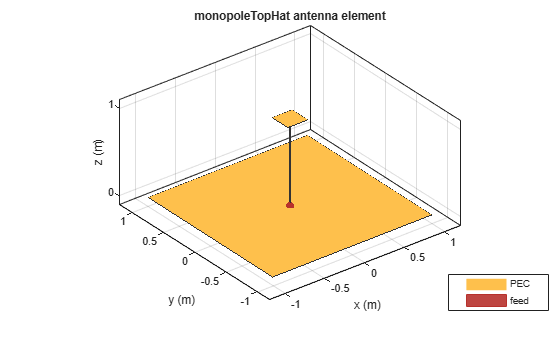Calculate and plot the impedance of a top-hat monopole over a frequency range of 40 MHz-80 MHz.

```th = monopoleTopHat; impedance(th,linspace(40e6,80e6,41));```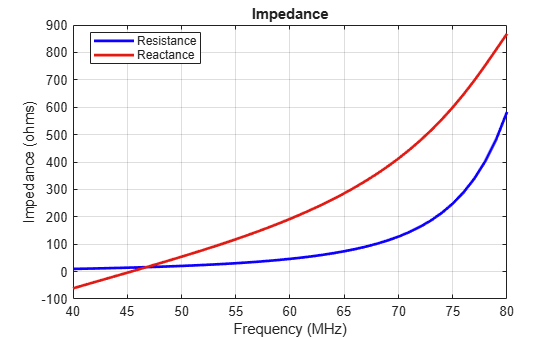Impedance comparison between a monopole of similar dimensions and the top-hat monopole in example 2.

```m = monopole; figure impedance(m,linspace(40e6,80e6,41));```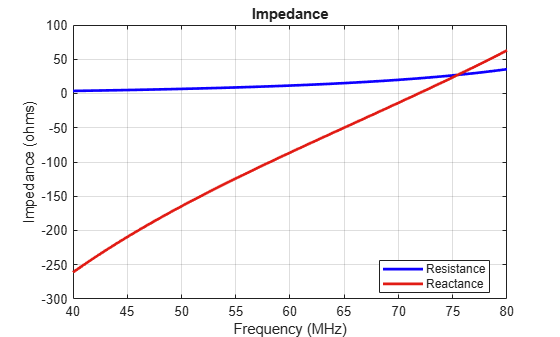Create a top-hat monopole with default dimensions and a substrate with two dielectric layers.

```mth = monopoleTopHat; d = dielectric('Name',{'FR4','Teflon'},'Thickness',[0.5 0.5],'LossTangent',[0.002 0.002],'EpsilonR',[4.8 2.1]); mth.Substrate = d```
```mth = monopoleTopHat with properties: Height: 1 Width: 0.0100 GroundPlaneLength: 2 GroundPlaneWidth: 2 TopHatLength: 0.2500 TopHatWidth: 0.2500 Substrate: [1x1 dielectric] FeedOffset: [0 0] Conductor: [1x1 metal] Tilt: 0 TiltAxis: [1 0 0] Load: [1x1 lumpedElement] ```

View the top-hat monopole antenna.

`show(mth)`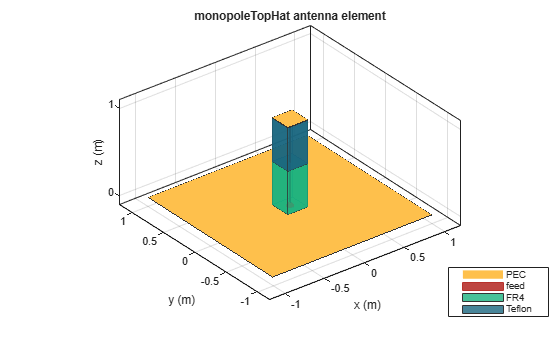Balanis, C.A. Antenna Theory. Analysis and Design, 3rd Ed. New York: Wiley, 2005.

 Volakis, John. Antenna Engineering Handbook, 4th Ed. New York: Mcgraw-Hill, 2007.